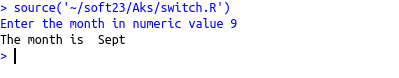• #5, First Floor, 4th Street Dr. Subbarayan Nagar Kodambakkam, Chennai-600 024 Landmark : Samiyar Madam
• pro@slogix.in
• +91- 81240 01111

### What is Decision making in R?

###### Description

To understand various decision making structures in R.

###### Process

Decision making structure :

• Block of codes are executed or skipped which is chosen by evaluating one or more test expression.In R there are
• If
• If….else
• If…elseif….else
• Switch

If statement :
Syntax

If(test expression)

{

Block of codes

<p}

If….else statement :
Syntax

If(test expression)

{

Block of codes

}else

{

Block of codes

}

If…elseif…else statement :
Syntax:

If(test expression)

{

Block of codes

}elseif

{

Block of codes

}else

{

Block of codes

}

NOTE: The else and elseif keyword should be given immediately after the braces.Failing this condition produces error

Switch statement :

• A particular value from the argument list is chosen according to a value(first argument)

Syntax
switch(exp,val1,val2,val3,……..)

###### Sapmle Code

Source code:(if…else)

#if else
if(x>y)
{
cat(“X is the greater than Y”)
}else
{
cat(“Y is greater than X”)
}

Source code :(if….elseif…else)

#if elseif else
units=as.integer(readline(prompt=”Enter the units consumed : “))
if(units<=100) { bill==0.00 cat(“No Eb charge.Totally Free\n”) }else if((units>100)&(units<=200)) { bill=20+((units-100)*1.50) }else if((units>200)&(units<=500))
{
bill=30+200+((units-200)*3.00)
}else
{
bill=50+350+1380+((units-500)*6.60)
}
cat(“Your EB bill is : “,bill)

Source code :(Switch statement)

x=as.integer(readline(prompt=”Enter the month in numeric value “))
y=switch(x,’Jan’,’Feb’,’Mar’,’Apr’,’May’,’June’,’July’,’Aug’,’Sept’,’Oct’,’Nov’,’Dec’)
cat(“The month is “,y)

###### Screenshots